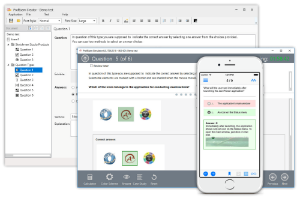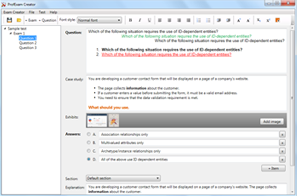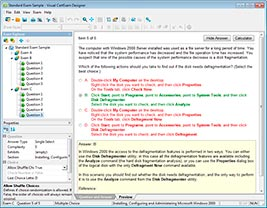# Download Lean Six Sigma Green Belt.prep4sure.LSSGB.2018-11-17.1e.97q.vcex

## File Info

 Exam Lean Six Sigma Green Belt Number LSSGB File Name Lean Six Sigma Green Belt.prep4sure.LSSGB.2018-11-17.1e.97q.vcex Size 458 Kb Posted November 17, 2018 Downloads 3

### How to open VCEX & EXAM Files?

Files with VCEX & EXAM extensions can be opened by ProfExam Simulator.

Coupon: EXAMFILESCOM
With discount: 20%

## Demo Questions

Question 1
The Regression Model for an observed value of Y contains the term β which represents the Y axis intercept when X = 0.

• A: True
• B: False

Question 2
Which statement(s) are true about the Fitted Line Plot shown here? (Note: There are 2 correct answers).

• A: When Reactant increases, the Energy Consumed increases.
• B: The slope of the equation is a positive 130.5.
• C: The predicted output Y is close to -18 when the Reactant level is set to 6.
• D: Over 85 % of the variation of the Energy Consumed is explained by the Reactant via this Linear Regression.

Question 3After reviewing the Capability Analysis shown here select the statement(s) that are untrue.

• A: The process is properly assumed to be a Normal process
• B: The Mean of the process moving range is 1.78
• C: The process is out of Control
• D: This Capability Analysis used subgroups
• E: Majority of the dimensional values are outside of the tolerance than within

Question 4
The actual experimental response data varied somewhat from what a Belt had predicted them to be. This is the result of which of these?

• A: Inefficiency of estimates
• B: Residuals
• C: Confounded data
• D: Gap Analysis

Question 5
What is the Cycle Time, in minutes, for a process having a Throughput of 360 units per hour?

• A: 0.167
• B: 0.333
• C: 0.667
• D: 1.333

Question 6
The generation of a Regression Equation is justified when we _____________.  (Note: There are 4 correct answers).

• A: Expect the relationship to be Linear between the output and inputs
• B: Know that there is a non-linear relationship between output and input(s)
• C: Need to understand how to control a process output by controlling the input(s)
• D: Experience several process defects and have no other way to fix hem
• E: When it is very expensive or too late to measure the output

Question 7
According to the definition of Rolled Throughput Yield which of the following items best describe the purpose of RTY?

• A: A function of Y=f(x)
• B: Determines incremental Growth
• C: Isolates the increase throughput
• D: Accounts for rejects and reworks

Question 8
The following Business Case is constructed properly.
“During fiscal year 2008 the warranty returns for electric razor Model 312 were 1.3%. This represents a gap of 0.5% over target costing the company \$18,500 per month.”

• A: True
• B: False

Question 9
Which statement(s) are true about the Fitted Line Plot shown here? (Note: There are 2 correct answers).

• A: When Reactant increases, the Energy Consumed increases.
• B: The slope of the equation is a positive 130.5.
• C: The predicted output Y is close to -18 when the Reactant level is set to 6.
• D: Over 85 % of the variation of the Energy Consumed is explained by the Reactant via this Linear Regression.

Question 10
Select all the statements that are true after reviewing the Capability Analysis shown here. (Note: There are 4 correct answers).

• A: The process is out of Control.
• B: The process is properly assumed to be a Normal process.
• C: The Mean of the process moving range is 1.78.
• D: This Capability Analysis used subgroups.
• E: Majority of the dimensional values are outside of the tolerance than within.

CONNECT US##### PROFEXAM WITH A 20% DISCOUNT

You can buy ProfExam with a 20% discount..

HOW TO OPEN VCEX AND EXAM FILES

Use ProfExam Simulator to open VCEX and EXAM filesHOW TO OPEN VCE FILES

Use VCE Exam Simulator to open VCE files